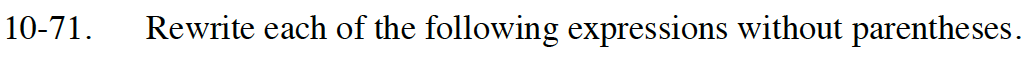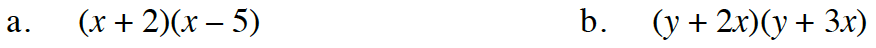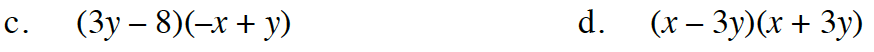### Home > INT1 > Chapter Ch10 > Lesson 10.1.4 > Problem10-71

10-71.
1. Rewrite each of the following expressions without parentheses. Homework Help ✎

1. (x + 2)(x – 5)

2. (y + 2x)(y + 3x)

3. (3y – 8)(–x + y)

4. (x – 3y)(x + 3y)Review the Math Notes box in section 3.2.2

Review the Math Notes box in section 3.2.2

y2 + 5xy + 6x2−3xy + 8x + 3y2 − 8y

Review the Math Notes box in section 3.2.2